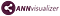# Visualizing the architecture of your own model of Artificial Neural Networks

This is a very simple post I’ve prepared just to help anyone who wants to visualize their artificial neural network architecture.

In this example, I will use a neural network built using Keras (http://keras.io/) and visualizing the architecture using ANN Visualizer (https://github.com/Prodicode/ann-visualizer).

The complete solution code can be found at:ANN visualizer — A great visualization python library used to work with Keras

You will need to install the following dependencies:

`\$ pip3 install keras\$ pip3 install ann_visualizer\$ pip install graphviz\$ pip install h5py`

Now just train the template used the script:

`# Create your first MLP in Kerasfrom keras.models import Sequentialfrom keras.layers import Denseimport numpy# fix random seed for reproducibilitynumpy.random.seed(7)# load pima indians datasetdataset = numpy.loadtxt("pima-indians-diabetes.data.csv", delimiter=",")# split into input (X) and output (Y) variablesX = dataset[:,0:8]Y = dataset[:,8]# create modelmodel = Sequential()model.add(Dense(12, input_dim=8, activation='relu'))model.add(Dense(8, activation='relu'))model.add(Dense(1, activation='sigmoid'))# Compile modelmodel.compile(loss='binary_crossentropy', optimizer='adam', metrics=['accuracy'])# Fit the modelmodel.fit(X, Y, epochs=150, batch_size=10)# evaluate the modelscores = model.evaluate(X, Y)print("\n%s: %.2f%%" % (model.metrics_names, scores*100))model_json = model.to_json()with open("model.json", "w") as json_file:    json_file.write(model_json)# serialize weights to HDF5model.save_weights("model.h5")print("Saved model to disk")`

Finally, you can view by invoking the ANN Visualizer from your saved template:

`from ann_visualizer.visualize import ann_viz;from keras.models import model_from_jsonimport numpy# fix random seed for reproducibilitynumpy.random.seed(7)# load json and create modeljson_file = open('model.json', 'r')loaded_model_json = json_file.read()json_file.close()model = model_from_json(loaded_model_json)# load weights into new modelmodel.load_weights("model.h5")ann_viz(model, title="Artificial Neural network - Model Visualization")`

The result is a file (.pdf) that will be saved in the project directory.

References:

Computer Engineer, Research Scientist in Artificial Intelligence

## More from João Gabriel Lima

Computer Engineer, Research Scientist in Artificial Intelligence